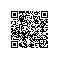# 关于Random

Random类中实现的随机算法是伪随机，也就是有规则的随机。在进行随机时，随机算法的起源数字称为种子数(seed)，在种子数的基础上进行一定的变换，从而产生需要的随机数字。

1、Random对象的生成
Random类包含两个构造方法，下面依次进行介绍：
a、public Random()

b、public Random(long seed)

Random r = new Random();
Random r1 = new Random(10);

2、Random类中的常用方法
Random类中的方法比较简单，每个方法的功能也很容易理解。需要说明的是，Random类中各方法生成的随机数字都是均匀分布的，也就是说区间内部的数字生成的几率是均等的。下面对这些方法做一下基本的介绍：
a、public boolean nextBoolean()

b、public double nextDouble()

c、public int nextInt()

d、public int nextInt(int n)

e、public void setSeed(long seed)

3、Random类使用示例

Random r = new Random();
a、生成[0,1.0)区间的小数
double d1 = r.nextDouble();

b、生成[0,5.0)区间的小数
double d2 = r.nextDouble() * 5;

c、生成[1,2.5)区间的小数
double d3 = r.nextDouble() * 1.5 + 1;

d、生成任意整数
int n1 = r.nextInt();

e、生成[0,10)区间的整数
int n2 = r.nextInt(10);
n2 = Math.abs(r.nextInt() % 10);

int n2 = r.nextInt(n);
n2 = Math.abs(r.nextInt() % n);
f、生成[0,10]区间的整数
int n3 = r.nextInt(11);
n3 = Math.abs(r.nextInt() % 11);

g、生成[-3,15)区间的整数
int n4 = r.nextInt(18) - 3;
n4 = Math.abs(r.nextInt() % 18) - 3;使用钉钉扫一扫加入圈子
+ 订阅